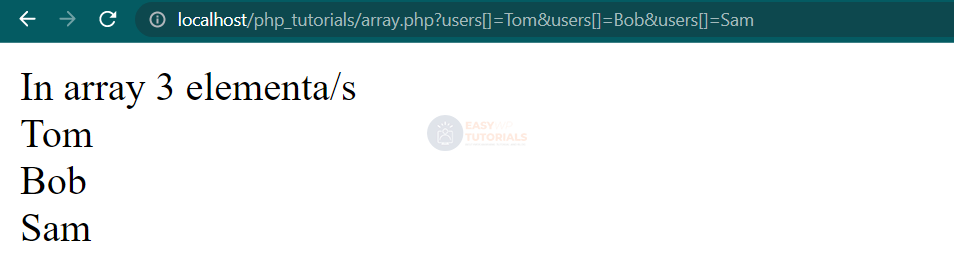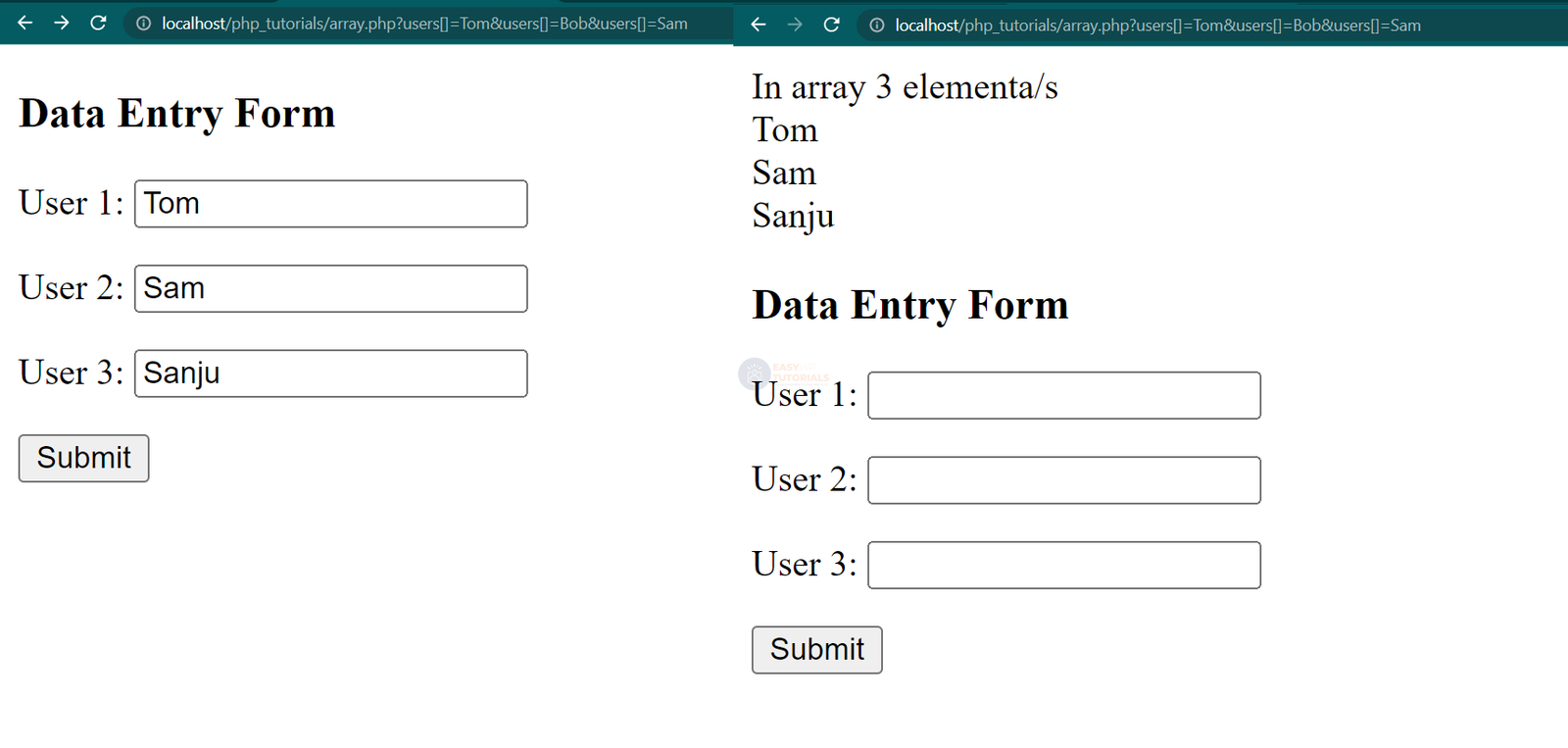# Sending arrays data in php

In previous topics, sending individual values ​​to the server was covered. However, sending a set of values, i.e. arrays, to PHP can be tricky. Consider how you can send to the server and, accordingly, receive arrays of data on the server.

For example, let’s define the following users.php file :

```<?php
\$users = [];
if (isset(\$_GET["users"])) {

\$users = \$_GET["users"];
}
echo "In array " . count(\$users) . " elementa/s<br>";
foreach (\$users as \$user) {
echo "\$user<br>";
};
?>
```

In this case, we assume that the “users” parameter, which is passed in a GET request, will be an array. And accordingly, we can get data from it.

To pass an array to this script, we’ll call it with the following query:

http://localhost/php_tutorials/array.php?users[]=Tom&users[]=Bob&users[]=Sam

To define a query string parameter as an array, square brackets [] follow the parameter name. We can then assign some value: users[]=Tom. And how many times will values be assigned in this way, so many ​​will be in the array? All values, as usual, are separated by an ampersand. So, in this case, three values ​​are passed to the collection.Similarly, we can send data in a POST request from a form. For example, let’s define the following script:

```<!DOCTYPE html>
<html>
<title>easywptutorials.com</title>
<meta charset="utf-8" />
<body>
<?php
if(isset(\$_POST["users"])){

\$users = \$_POST["users"];
echo "In array " . count(\$users) . " elementa/s<br>";
foreach(\$users as \$user) echo "\$user<br>";
}
?>
<h3>Data Entry Form</h3>
<form method="POST">
<p>User 1: <input type="text" name="users[]" /></p>
<p>User 2: <input type="text" name="users[]" /></p>
<p>User 3: <input type="text" name="users[]" /></p>
<input type="submit" value="Отправить">
</form>
</body>
</html>
```

As you know, the name of the key of the data transmitted to the server corresponds to the value of the attribute name of the form element. And to indicate that some input field will supply a value to an array, the name input field attribute takes the name of the array with square brackets as its value:

```<input type="text" name="users[]" />
```

Accordingly, how many input fields with the same array name do we specify, so many values ​​we can transfer to the server? So, in this case, three values ​​are passed to the server in the user’s array:Moreover, this principle applies to other types of input fields in the html form.

At the same time, in the examples above, a regular array was passed, each element of which has a numeric index as a key. Accordingly, using the index, we can get a specific element of the array:

```
\$firstUser = \$_POST["users"];
echo \$firstUser;
```

But we can also explicitly specify the keys in the form elements:

```<!DOCTYPE html>
<html>
<title>easywptutorials.com</title>
<meta charset="utf-8" />
<body>
<?php
if(isset(\$_POST["users"])){

\$firstUser = \$_POST["users"]["first"];
\$secondUser = \$_POST["users"]["second"];
\$thirdUser = \$_POST["users"]["third"];
echo "\$firstUser<br>\$secondUser<br>\$thirdUser";
}
?>
<h3>Data Entry Form</h3>
<form method="POST">
<p>User 1: <input type="text" name="users[first]" /></p>
<p>User 2: <input type="text" name="users[second]" /></p>
<p>User 3: <input type="text" name="users[third]" /></p>
<input type="submit" value="Submit">
</form>
</body>
</html>
```

For example, the first field adds an element with the key “first” to the array

```<input type="text" name="users[first]" />
```

Therefore, on the server, we can use this key to get the corresponding element:

```
\$firstUser = \$_POST["users"]["first"];
```